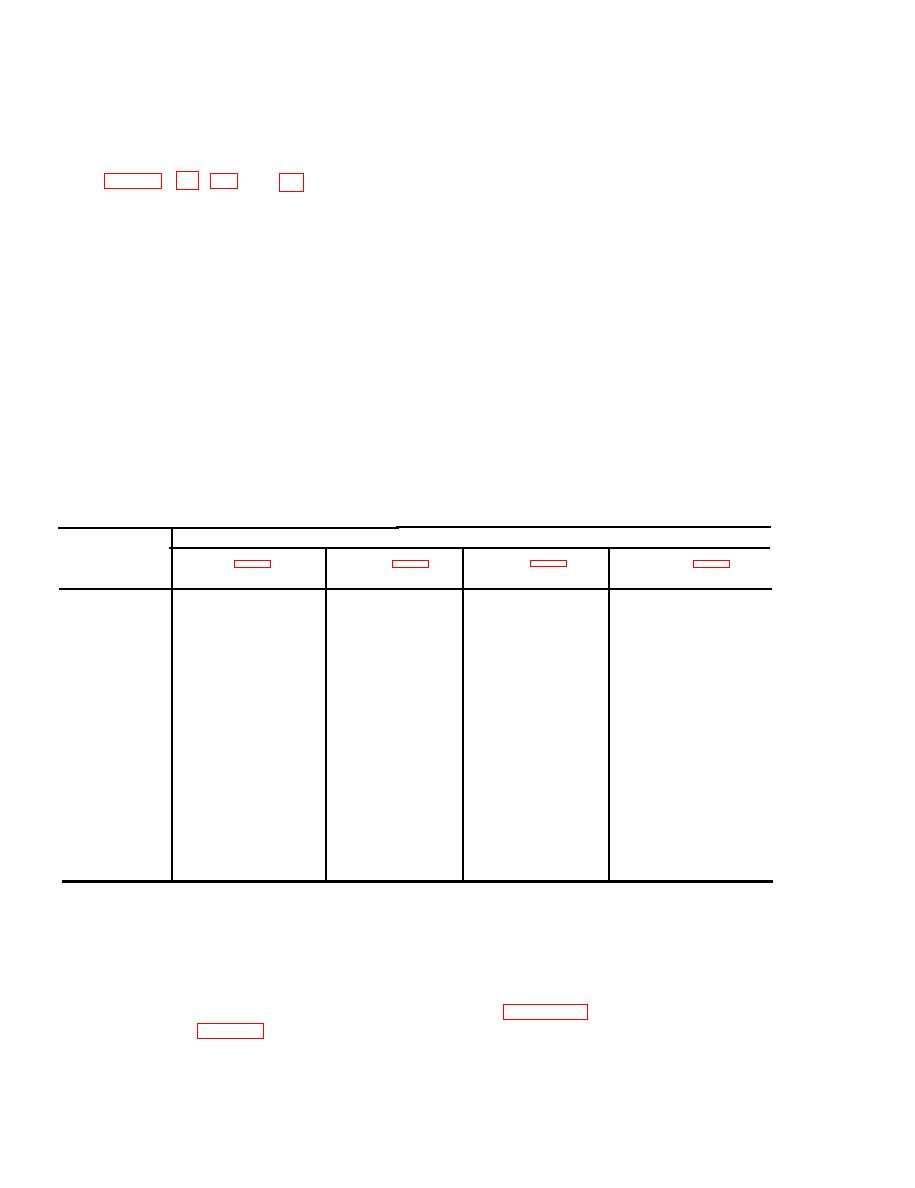Click here to make tpub.com your Home Page Page Title: Dc Resistances of Tuning Unit Multi pin Connectors Back | Up | NextWeb www.tpub.comHome

Information Categories
Aerographer
Automotive
Aviation
Construction
Diving
Draftsman
Engineering
Electronics
Food and Cooking
Logistics
Math
Medical
Music
Nuclear Fundamentals
Photography
Religion( 3 ) For tuning unit 4, take measure-
68. Dc Resistances of Tuning Unit
ments with FREQUENCY RANGE-
Multi pin Connectors
MC switch S503 at the 400-700-mc
a. General.  A convenient set of test
position.
p o i n t s for resist a n c e measurements
N o t e : For tuning unit 3, take measure-
i s accessible at the rear of each tuning
ments at any frequency dial indication.
u n i t (fig. 56, 64, 68, and 72). With the
b. Chart of Resistance Measurements.
tuning unit r e m o v e d from the tuning
The chart below lists the resistance meas-
u n i t compartment of the main unit, the
u r e m e n t s at multipin connectors at the
m u 1 t i p i n connector permits a check-
rear of the tuning units under the following
out of 20 circuit points. M a k e measure-
conditions:
ments from each terminal of the connector
(1) The tuning unit removed from main
to tuning chassis ground. In a d d i t i o n
unit.
t o the general conditions of measurement
(2) T h e t e s t h a r n e s s d i s c o n n e c t e d
s h o w n in the chart below, set the band
f r o m receptacle at rear of tuning
switch on the applicable tuning unit as fol-
unit .
lows :
( 1 ) For tuning unit 1, take measure-
(3) The GAIN and SLIDE BACK con-
m e n t s with MEGACYCLES band
t r o l s in extreme counterclockwise
s w i t c h S1 at the .15- .36 (band 1)
position.
position.
(4) All measurements taken from des-
( 2 ) For tuning unit 2, take measure-
ignated terminal to tuning unit
ments with FREQUENCY RANGE-
c h a s s i s ground.
M C switch S201 at the 20-70-mc
(5) The mating pin numbers for tuning
position.
units 1 through 4 indicated on P1.
Resistance (ohms)
Terminal
(Tuning unit 4)
(Tuning unit 3)
(Tuning unit 2)
(Tuning unit 1)
Infinity
Infinity
Infinity
A1
Infinity
0
0
0
A2
0.8
50K
50 K
55K
1
38K
Infinity
Infinity
2
6K
Infinity
Infinity
Infinity
Iinfinity
3
Infinity
0
0
0
4
0
Infinity
Infinity
Infinity
Infinity
5
Infinity
Infinity
Infinity
6
Infinity
Infinity
Infinity
Infinity
7
Infinity
Infinity
Infinity
Infinity
8
Infinity
Infinity
Infinity
Infinity
9
Infinity
Infinity
Infinity
Infinity
Infinity
10
Infinity
Infinity
Infinity
Infinity
11
Infinity
Infinity
Infinity
Infinity
12
0
0
0
0
13
30K
30K
30 K
14
30K
30K
30K
30K
15
30K
0.8
0.8
0.8
16
1.8
0.8
0.8
1.6
0.8
17
320
310
320
18
320
formers mounted in the various if. pre-
69. Dc Resistances of Transformers
amplifier and if. amplifier subassemblies
and Coils
do not have terminal numbers stamped on
a. General. The dc resistance of trans-
t h e phenolic wafer at the bottom of the
f o r m e r windings and coils is listed in c
s h i e l d can. Therefore, reference must be
below.
made to figure 77 for identifying which
b. Key to Identifying Terminals on If.
terminals are for the primary winding and
T r a n s f o r m e r s (fig. 77). The if. trans-
152Integrated Publishing, Inc. - A (SDVOSB) Service Disabled Veteran Owned Small Business